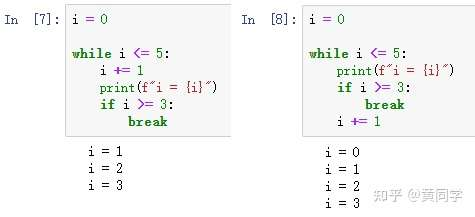﻿ Python基础 while循环与break、continue关键字_python_脚本之家
python# Python基础 while循环与break、continue关键字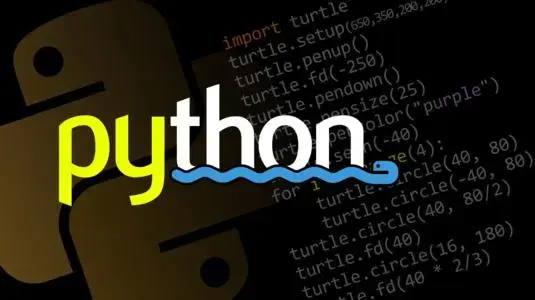`Python`中for循环和while循环本质上是没有区别的，但是在实际应用上，针对性不太一样。

• for循环，主要应用在遍历中，体现的是遍历二字；
• `while`循环，主要用于判断符合条件下循环，体现的是条件二字；

## 1. while循环语法结构

`while`循环，表示程序一直运行，直到指定的条件不再满足时，就终止程序。while循环的语法结构如下：

```while 判断条件(condition)：
执行代码块儿(statements)……

```

## 2. +=和-=用做退出条件### 2.1 +=用做退出条件

```counts = 1

while counts <= 5:
print("我吃饱了！")
counts += 1
```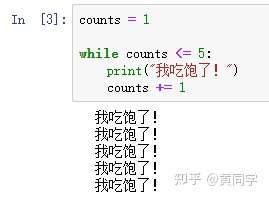### 2.2 -=用做退出条件

```counts = 5

while counts > 0:
print("我好饿！")
counts -= 1
```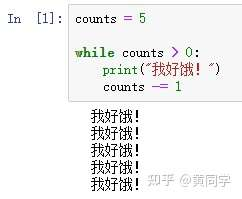## 3. break和continue关键字

`Python`中，有`continue``break`两个关键字，供我们使用。记住一点：`continue``break`两个关键字，都可以结合`for`循环和`while`循环来使用。

### 3.2 continue结合for循环和while循环使用

① continue + for循环

```for i in range(5):
if i == 3:
print("continue之前的代码，会执行")
continue
print("continue之后的代码，不会执行")

```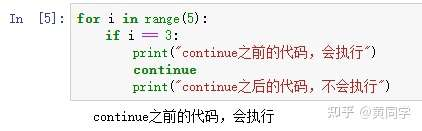② continue + while循环

```i = 5

while i > 0:
i -= 1
if i >= 3:
print("我在continue之前，会执行")
continue
print("我在continue之后，不会执行")
```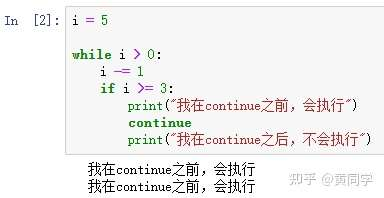### 3.3 break结合for循环和while循环使用

`break`就比较狠了，直接终止循环。

① break + for循环

```for i in range(5):
print(f"i = {i}")
if i >= 3:
break

```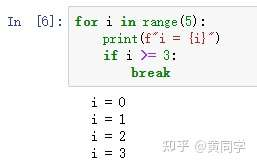② break + while循环

```i = 0

while i <= 5:
i += 1
print(f"i = {i}")
if i >= 3:
break
-----------------------
i = 0

while i <= 5:
print(f"i = {i}")
if i >= 3:
break
i += 1
```